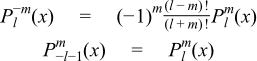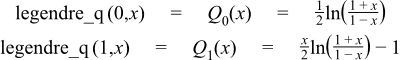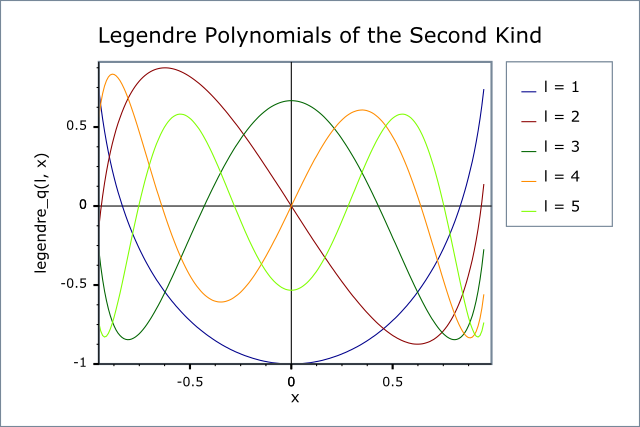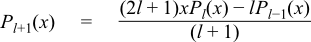#Boost C++ Libraries

...one of the most highly regarded and expertly designed C++ library projects in the world.

This is the documentation for an old version of boost. Click here for the latest Boost documentation.

#### Legendre (and Associated) Polynomials

##### Synopsis

```#include <boost/math/special_functions/legendre.hpp>
```

```namespace boost{ namespace math{

template <class T>
calculated-result-type legendre_p(int n, T x);

template <class T, class Policy>
calculated-result-type legendre_p(int n, T x, const Policy&);

template <class T>
calculated-result-type legendre_p(int n, int m, T x);

template <class T, class Policy>
calculated-result-type legendre_p(int n, int m, T x, const Policy&);

template <class T>
calculated-result-type legendre_q(unsigned n, T x);

template <class T, class Policy>
calculated-result-type legendre_q(unsigned n, T x, const Policy&);

template <class T1, class T2, class T3>
calculated-result-type legendre_next(unsigned l, T1 x, T2 Pl, T3 Plm1);

template <class T1, class T2, class T3>
calculated-result-type legendre_next(unsigned l, unsigned m, T1 x, T2 Pl, T3 Plm1);

}} // namespaces
```

The return type of these functions is computed using the result type calculation rules: note than when there is a single template argument the result is the same type as that argument or `double` if the template argument is an integer type.

The final Policy argument is optional and can be used to control the behaviour of the function: how it handles errors, what level of precision to use etc. Refer to the policy documentation for more details.

##### Description
```template <class T>
calculated-result-type legendre_p(int l, T x);

template <class T, class Policy>
calculated-result-type legendre_p(int l, T x, const Policy&);
```

Returns the Legendre Polynomial of the first kind:Requires -1 <= x <= 1, otherwise returns the result of domain_error.

Negative orders are handled via the reflection formula:

P-l-1(x) = Pl(x)

The following graph illustrates the behaviour of the first few Legendre Polynomials:```template <class T>
calculated-result-type legendre_p(int l, int m, T x);

template <class T, class Policy>
calculated-result-type legendre_p(int l, int m, T x, const Policy&);
```

Returns the associated Legendre polynomial of the first kind:Requires -1 <= x <= 1, otherwise returns the result of domain_error.

Negative values of l and m are handled via the identity relations:CautionThe definition of the associated Legendre polynomial used here includes a leading Condon-Shortley phase term of (-1)m. This matches the definition given by Abramowitz and Stegun (8.6.6) and that used by Mathworld and Mathematica's LegendreP function. However, uses in the literature do not always include this phase term, and strangely the specification for the associated Legendre function in the C++ TR1 (assoc_legendre) also omits it, in spite of stating that it uses Abramowitz and Stegun as the final arbiter on these matters. See: Abramowitz, M. and Stegun, I. A. (Eds.). "Legendre Functions" and "Orthogonal Polynomials." Ch. 22 in Chs. 8 and 22 in Handbook of Mathematical Functions with Formulas, Graphs, and Mathematical Tables, 9th printing. New York: Dover, pp. 331-339 and 771-802, 1972.
```template <class T>
calculated-result-type legendre_q(unsigned n, T x);

template <class T, class Policy>
calculated-result-type legendre_q(unsigned n, T x, const Policy&);
```

Returns the value of the Legendre polynomial that is the second solution to the Legendre differential equation, for example:Requires -1 <= x <= 1, otherwise domain_error is called.

The following graph illustrates the first few Legendre functions of the second kind:```template <class T1, class T2, class T3>
calculated-result-type legendre_next(unsigned l, T1 x, T2 Pl, T3 Plm1);
```

Implements the three term recurrence relation for the Legendre polynomials, this function can be used to create a sequence of values evaluated at the same x, and for rising l. This recurrence relation holds for Legendre Polynomials of both the first and second kinds.For example we could produce a vector of the first 10 polynomial values using:

```double x = 0.5;  // Abscissa value
vector<double> v;
v.push_back(legendre_p(0, x)).push_back(legendre_p(1, x));
for(unsigned l = 1; l < 10; ++l)
v.push_back(legendre_next(l, x, v[l], v[l-1]));
```

Formally the arguments are:

l

The degree of the last polynomial calculated.

x

The abscissa value

Pl

The value of the polynomial evaluated at degree l.

Plm1

The value of the polynomial evaluated at degree l-1.

```template <class T1, class T2, class T3>
calculated-result-type legendre_next(unsigned l, unsigned m, T1 x, T2 Pl, T3 Plm1);
```

Implements the three term recurrence relation for the Associated Legendre polynomials, this function can be used to create a sequence of values evaluated at the same x, and for rising l.For example we could produce a vector of the first m+10 polynomial values using:

```double x = 0.5;  // Abscissa value
int m = 10;      // order
vector<double> v;
v.push_back(legendre_p(m, m, x)).push_back(legendre_p(1 + m, m, x));
for(unsigned l = 1 + m; l < m + 10; ++l)
v.push_back(legendre_next(l, m, x, v[l], v[l-1]));
```

Formally the arguments are:

l

The degree of the last polynomial calculated.

m

The order of the Associated Polynomial.

x

The abscissa value

Pl

The value of the polynomial evaluated at degree l.

Plm1

The value of the polynomial evaluated at degree l-1.

##### Accuracy

The following table shows peak errors (in units of epsilon) for various domains of input arguments. Note that only results for the widest floating point type on the system are given as narrower types have effectively zero error.

Table 29. Peak Errors In the Legendre P Function

Significand Size

Platform and Compiler

Errors in range

0 < l < 20

Errors in range

20 < l < 120

53

Win32, Visual C++ 8

Peak=211 Mean=20

Peak=300 Mean=33

64

SUSE Linux IA32, g++ 4.1

Peak=70 Mean=10

Peak=700 Mean=60

64

Red Hat Linux IA64, g++ 3.4.4

Peak=70 Mean=10

Peak=700 Mean=60

113

HPUX IA64, aCC A.06.06

Peak=35 Mean=6

Peak=292 Mean=41

Table 30. Peak Errors In the Associated Legendre P Function

Significand Size

Platform and Compiler

Errors in range

0 < l < 20

53

Win32, Visual C++ 8

Peak=1200 Mean=7

64

SUSE Linux IA32, g++ 4.1

Peak=80 Mean=5

64

Red Hat Linux IA64, g++ 3.4.4

Peak=80 Mean=5

113

HPUX IA64, aCC A.06.06

Peak=42 Mean=4

Table 31. Peak Errors In the Legendre Q Function

Significand Size

Platform and Compiler

Errors in range

0 < l < 20

Errors in range

20 < l < 120

53

Win32, Visual C++ 8

Peak=50 Mean=7

Peak=4600 Mean=370

64

SUSE Linux IA32, g++ 4.1

Peak=51 Mean=8

Peak=6000 Mean=480

64

Red Hat Linux IA64, g++ 3.4.4

Peak=51 Mean=8

Peak=6000 Mean=480

113

HPUX IA64, aCC A.06.06

Peak=90 Mean=10

Peak=1700 Mean=140

Note that the worst errors occur when the order increases, values greater than ~120 are very unlikely to produce sensible results, especially in the associated polynomial case when the degree is also large. Further the relative errors are likely to grow arbitrarily large when the function is very close to a root.

No comparisons to other libraries are shown here: there appears to be only one viable implementation method for these functions, the comparisons to other libraries that have been run show identical error rates to those given here.

##### Testing

A mixture of spot tests of values calculated using functions.wolfram.com, and randomly generated test data are used: the test data was computed using NTL::RR at 1000-bit precision.

##### Implementation

These functions are implemented using the stable three term recurrence relations. These relations guarentee low absolute error but cannot guarentee low relative error near one of the roots of the polynomials.